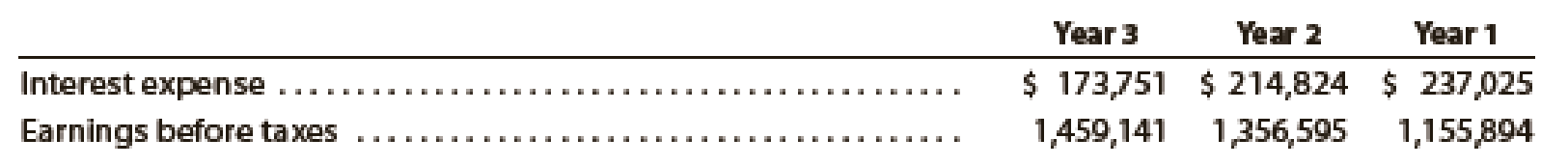# The following financial data (in thousands) were taken from recent financial statements of Staples, Inc. : 1. Determine the times interest earned ratio for Staples in Year 3, Year 2, and Year 1? Round your answers to one decimal place. 2. Evaluate this ratio for Staples.### Financial Accounting

15th Edition
Carl Warren + 2 others
Publisher: Cengage Learning
ISBN: 9781337272124

#### Solutions

Chapter
Section### Financial Accounting

15th Edition
Carl Warren + 2 others
Publisher: Cengage Learning
ISBN: 9781337272124
Chapter 14, Problem 7CP
Textbook Problem
5 views

## The following financial data (in thousands) were taken from recent financial statements of Staples, Inc.:1. Determine the times interest earned ratio for Staples in Year 3, Year 2, and Year 1? Round your answers to one decimal place. 2. Evaluate this ratio for Staples.

1.

To determine

Calculate times-interest-earned ratio for the Incorporation S in Year 1, Year 2, and Year 3.

### Explanation of Solution

Times-Interest-Earned ratio: It is the ratio that quantifies a business ability to pay interest expense. It is calculated as shown below:

Times-interest-earned ratio}=Incomebeforeincometax+Interest expenseInterest expense

Determine the times-interest-earned ratio for the Incorporation S in Year 3, Year 2, and Year 1.

Year 3:

Earnings before taxes = $1,459,141 Interest Expense =$176,751

Times-interest- earned ratio}=Earningsbeforetaxes+Interest expenseInterest expense=$1,459,141+$173,751\$173,751=9

2.

To determine

Evaluate these ratios for Incorporation S.

### Still sussing out bartleby?

Check out a sample textbook solution.

See a sample solution

#### The Solution to Your Study Problems

Bartleby provides explanations to thousands of textbook problems written by our experts, many with advanced degrees!

Get Started